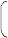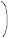## Terms related to Profit and Loss

• Cost price (C.P): Price at which an item is purchased.
• Selling price (S.P): Price at which an item is sold.
• Profit: When the selling price is greater than the cost price, it is called profit.
• Loss: When the selling price is less than the cost price, it is called as loss.
• Profit or loss per cent: Profit or Loss after selling an item of Rs. 100(C.P).
• Discount: Discount is the reduction in the market price.
• Market Price: Retailer marks the price on a label and attaches it to the articles.

## Profit & Loss Calculation

### Profit Calculation

Profit or Gain = selling price (S.P) – cost price (C.P)

Example :

S.P = 150, C.P = 20

Profit OR Gain = (150-120) = 30

### Loss Calculation

Loss = Cost price(C.P) – Selling price(S.P)

Example :

C.P = 100, S.P = 90

Loss = (100-90) = 10

Note: Profit or Loss per cent is always calculated on cost price(C.P).

## Formulas to calculate Profit & Loss

 (i) % of Profit =Profit x 100Cost price
 (ii) % of Loss =Loss x 100Cost price

(iii) In case of profit:

 S.P = C.P100 + % of profit100
 C.P =S.P x 100100 + % of profit

(iv) In case of Loss:

 S.P = C.P100 – % of Loss100
 C.P =S.P x 100100 – % of Loss

Related Topics: